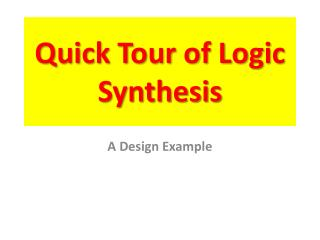DownloadDownload PresentationQuick Tour of Logic Synthesis

# Quick Tour of Logic Synthesis

Download Presentation## Quick Tour of Logic Synthesis

- - - - - - - - - - - - - - - - - - - - - - - - - - - E N D - - - - - - - - - - - - - - - - - - - - - - - - - - -
##### Presentation Transcript

1. Quick Tour of Logic Synthesis A Design Example

2. Outline • Simple Case Conversion Circuit • First Refinement • The Transform Block • The Command Interpreter • Technology Mapping

3. The LUNC Circuit LUNC = “Lower case, Upper case, No change, or “Change case” case conversion circuit Case conversion Circuit Simple CC

4. LUNC “Interface” Input: 8-bit ASCII code of an alphanumeric character Output: Case conversion of the input character: ^[L => convert to Lower case ^[U => convert to Upper case ^[N => No conversion ^[C => Change case Simple CC

5. LUNC Specification Input string: a b C d E f ^[ U a b C D … Output string: ? ? a b C d E f ? ? A B C D … ^[L => convert to Lower case ^[U => convert to Upper case ^[N => No conversion ^[C => Change case ? => don’t care Latency = 2 Simple CC

6. ASCII Codes and operation of change Hex Note Only change is in bit 5, so Code(a) = Code(A) +32 Simple CC

7. 2 registers in datapath, so latency is 2 cycles Controller FSM LUNC Block Diagram Refinement

8. Transform Block Behavioral Description ofcombinationallogic: ProcedureTRANSFORM (Rin,Lcmd,Ucmd,Ncmd,Ccmd) { if(Lcmd) {mux = TOLOWER(Rin)} else if(Ucmd) {mux = TOUPPER(Rin)} else if(Ncmd) {mux} = Rin} else if(Ccmd) {mux} = CHANGECASE(Rin) return(mux) } Transform Block

9. Transform Block • Remarks • A relatively high level description is easy to read and write • Calling the output MUX suggests that we can translate the IF-THEN-ELSE statement into a multiplexer Transform Block

10. Transform Block Diagram lower upper Simple translation Change case Transform Block

11. Transform Block Diagram • This implementation is clearly correct, but inelegant, since LC, UC, CC done every cycle • Synthesis tool reduces the chip areafrom 606 literals to 12 literals,if the don’t care conditions are specified • Then we show that a clever,but not so obviously correct,design can implement this function in 12 literalsdirectly. Transform Block

12. The ChangeCase Block Procedure CHANGECASE(Rin) { if (isUC(Rin)) {res = Rin + 32} else {res = Rin - 32} return (res) } Rin isUC / 8 res +/- 32 / 8 Transform Block

13. The Optimized Transform Block 0-00 => 0 (UC) 1-00 => Rin5 0-10 => Rin5’ 0-01 => 1 (LC) Out Modulo the “1-hot” don’t cares, script.rugged gets Out = NcmdRin5+L+CcmdRin5’, costing 5 lts (+7 “feedthrough” buffer lits makes 12) Note Ucmd is implicit 0-00 => Ucmd=1 Transform Block

14. The Command Interpreter Decoder: If previous character is escape, and if Current input character is L,U,N, or C, then the outputs Lcmd, Ucmd,Ncmd, and Ccmd are reset. Else they are Don’t Cares Register Latches Decoder Command Interpreter

15. The Command Interpreter Procedure lunc? (Rin) { if(Rin = L) Lcmd=1, Ucmd=Ncmd=Ccmd=0 else if (Rin = U) Ucmd=1, Lcmd=Ncmd=Ccmd=0 else if (Rin = N) Ncmd=1, Lcmd=Ucmd=Ccmd=0 else if (Rin = C) Ccmd=1, Lcmd=Ucmd=Ccmd=0 else Lcmd=Ucmd=Ncmd=Ccmd=Don't~Care return (Lcmd, Ucmd, Ncmd, Ccmd) } Command Interpreter

16. Comparison For Equality A=B A 7 B 7 A=escape=0x1b=27 A 7 A 0 A 0 B 0

17. Two Least significant bits A1 A0 Distinguishing L,U,N,C L = 0x4C = 01001100 = 74 U = 0x55 = 01010101 = 85 N = 0x4E = 01001110 = 78 C = 0x43 = 01000011 = 67 The last2 bits are sufficient for distinguishing L,U,N,C Everything else is don’t care Note isC not needed because of the “One Hot” assumption Command Interpreter

18. 0 > 0 > 0 > 1 0 1 0 1 0 Optimized Command Interpreter in7,…,in1,in0 MUXs choose between current isL, isN, isC and previous state isESC isL oL Delay of 1 isN oN 1 > isC oC Command Interpreter

19. TB Only isEsc is delayed Overall Latency of 2 LUNC x7 x0 o7 o0 i7 i0 b5 reg8 x5 reg8 x7,…x1,x0 Lcmd Ncmd Ccmd CI Command Interpreter

20. B 0 1 0 0 0 1 1 0 f 0 1 1 0 0 1 1 0 ? 0 1 1 0 0 1 1 0 A 0 1 0 0 0 0 0 1 ? 0 1 1 0 0 1 1 0 Esc:00011011, U:01010101, 1, 1 0, 0, a:01100001, b:01100010 0, 1, 1, 0 1, 0, 0, 0 0, 1, 0, 0 Ncmd switches from 1 to 0 on 10th clock tick Final LUNC E 0 1 0 0 0 1 0 1 Clock Tick: t=7, 8, 9, 10, 11 input: I=^[, U, a, b, C b5 7 8 9 10 11 f:01100110, TB x5=1, b5=1, 7,8, 9,10,11 isESC:0, Lcmd 0, Ncmd Ccmd LUNC Simulation CI Command Interpreter

21. Technology Mapping • Synthesis • Translation • Optimization • Technology mapping • Technology dependent • Technology independent Technology Mapping

22. Quick Tour of Logic Synthesis Sungho Kang Yonsei University